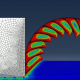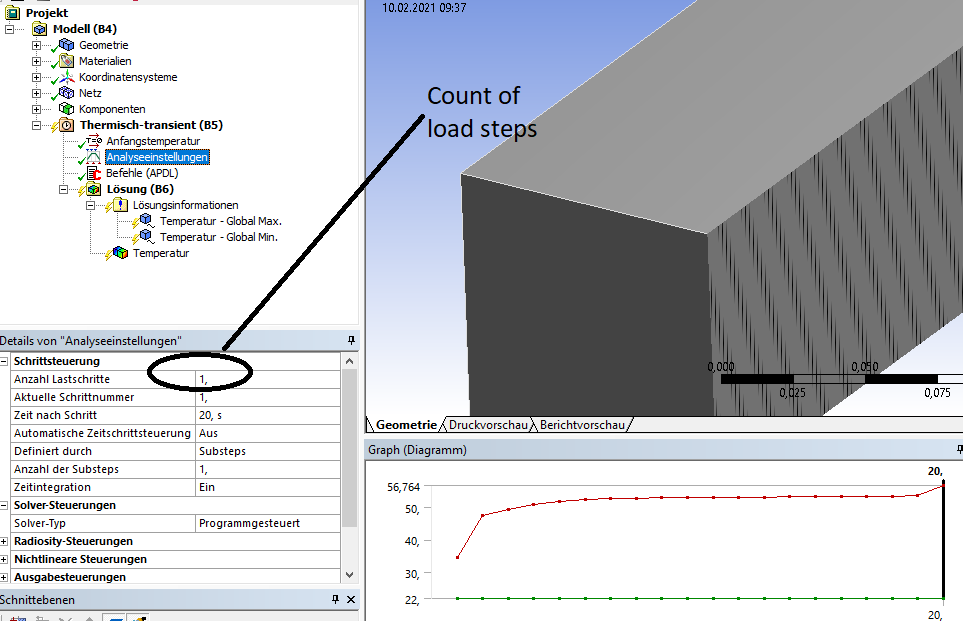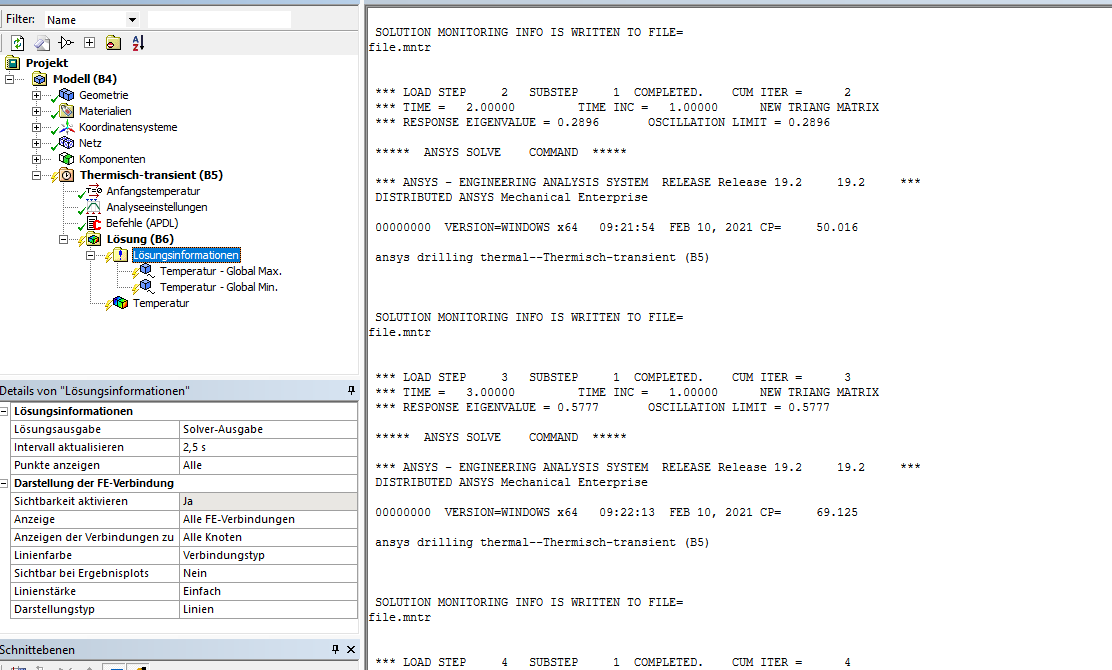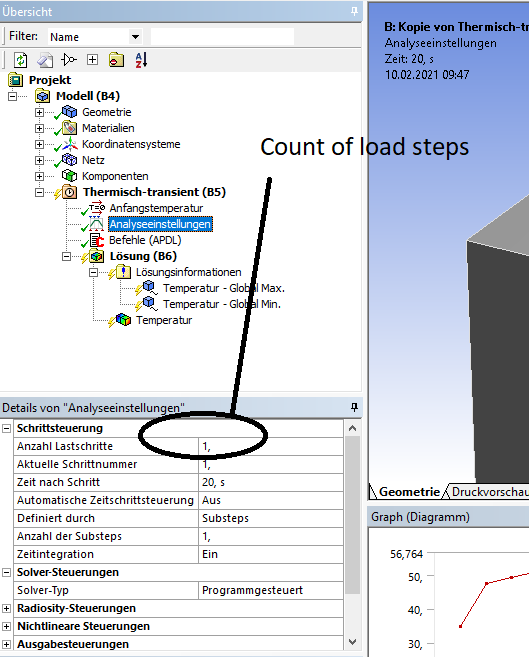## General Mechanical

•ANSYS_FEM_Guy
Subscriber

Hello,

I'm trying to implement Element kill in my analysis.

It's a simple simulation with a moving vomumetric heat source.

•1shan
Ansys Employee
,nPlease check this link https://simutechgroup.com/performing-ekill-element-death-in-ansys-workbench-mechanical-mdoel/ and let me know if it helps.nnRegards,nIshan.n
•ANSYS_FEM_Guy
Subscriber
Hello @1shan,nthank you for your answer. I tried to implement the ekill snippet in my apdl block, but it's not working.nCMSEL,ALLn*GET,EMAX,ELEM,,NUM,MAX     n*GET,EMIN,ELEM,,NUM,MIN     nALLSELQ=4e06                                                    nPI=acos(-1)nd=0.02nr_spot=0.020time_weld=20                     ntime_inc=1                         ntime_steps=time_weld/time_inc  nVEL=0.01                       *DO,i,1,time_steps,1              nWTIME=(i*time_inc)nTIME,WTIME         *DO,jj,EMIN,EMAX,1                 nX=CENTRX(jj)                       nY=CENTRY(jj)                       nZ=CENTRZ(jj)                       CSI_Z=-Z-WTIME*VELnCSI_X=XnCSI_Y=Ynr=sqrt((CSI_X)**2+(CSI_Y)**2)dT=CSI_Z+d*IF,Z,LT,CSI_Z,THENnQU=0n*ELSEIF,Z,GT,dT,THENnQU=0n*ELSEIF,r,GT,r_spot,THENnQU=0n*ELSEnQU=Q/(r_spot**2+PI*d)n*ENDIFn                                                      nBFE,jj,HGEN,,QU                           n*ENDDOnSOLVEn*ENDDOCMSEL,ALL                                 nBFEDELE,ALL,ALL                           nALLSEL/com,n/com, n*get,myncmls,active,,solu,ncmls                       ! cumulative number of load stepsn*if,myncmls,gt,0,then                                 ! if this is after the firstncmsel,s,elem_to_kill_body                             ! ELEMENT component to be checkednnsle                                                  ! nodes on these elementsnSELTOL,1.0e-12                                        ! stay within range belownnsel,r,bf,temp,50                                     ! re-select nodes with results above a value <<I apply the load within one step.nI'm not sure how to implement the ekill properly in a way that's compatible with my code.nnBest regardsnSam Donell
•ANSYS_FEM_Guy
Subscriber
Hello ,nthank you for the answer. I implemented the ekill snippet in my code but it's still not working.nCMSEL,ALLn*GET,EMAX,ELEM,,NUM,MAX     n*GET,EMIN,ELEM,,NUM,MIN     nALLSELQ=4e06                                                    nPI=acos(-1)nd=0.02nr_spot=0.020time_weld=20                     ntime_inc=1                         ntime_steps=time_weld/time_inc  nVEL=0.01                       *DO,i,1,time_steps,1              nWTIME=(i*time_inc)nTIME,WTIME         *DO,jj,EMIN,EMAX,1                 nX=CENTRX(jj)                       nY=CENTRY(jj)                       nZ=CENTRZ(jj)                       CSI_Z=-Z-WTIME*VELnCSI_X=XnCSI_Y=Ynr=sqrt((CSI_X)**2+(CSI_Y)**2)dT=CSI_Z+d*IF,Z,LT,CSI_Z,THENnQU=0n*ELSEIF,Z,GT,dT,THENnQU=0n*ELSEIF,r,GT,r_spot,THENnQU=0n*ELSEnQU=Q/(r_spot**2+PI*d)n*ENDIFn                                                      nBFE,jj,HGEN,,QU                           n*ENDDOnSOLVEn*ENDDOCMSEL,ALL                                 nBFEDELE,ALL,ALL                           nALLSEL/com,n/com, n*get,myncmls,active,,solu,ncmls                       ! cumulative number of load stepsn*if,myncmls,gt,0,then                                 ! if this is after the firstncmsel,s,elem_to_kill_body                             ! ELEMENT component to be checkednnsle                                                  ! nodes on these elementsnSELTOL,1.0e-12                                        ! stay within range belownnsel,r,bf,temp,50                                     ! re-select nodes with results above a value <<Best regards nSam Donellnn